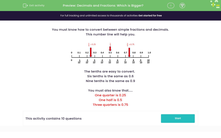# Convert Fractions to Decimals to Find the Bigger or Smaller Value

In this worksheet, students will convert between simple fractions and decimals to decide which of two given fractions is bigger or smaller.Key stage:  KS 2

Curriculum topic:   Number: Fractions and Decimals

Curriculum subtopic:   Recognise Decimal Equivalents

Popular topics:   Fractions worksheets

Difficulty level:#### Worksheet Overview

We need to know how to convert between simple fractions and decimals.The tenths are easy to convert.

Six tenths is the same as 0.6

Nine tenths is the same as 0.9

We also need to know that:

One quarter is 0.25

One half is 0.5

Three quarters is 0.75Let's have a go at some questions now.

### What is EdPlace?

We're your National Curriculum aligned online education content provider helping each child succeed in English, maths and science from year 1 to GCSE. With an EdPlace account you’ll be able to track and measure progress, helping each child achieve their best. We build confidence and attainment by personalising each child’s learning at a level that suits them.

Get started#### Popular Maths topics

••••